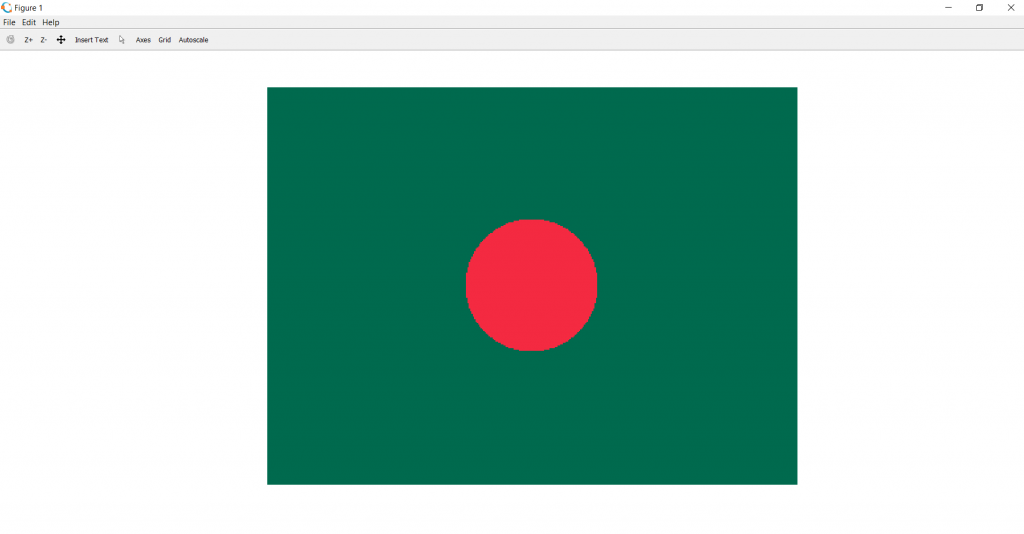# Draw Bangladesh Flag Using Matlab

Prerequisite: RGB image representation
A colored image can be represented as a 3 order matrix. The first order is for the rows, the second order is for the columns and the third order is for specifying the color of the corresponding pixel. Here we use the RGB color format, so the third order will take 3 values of Red, Green ans Blue respectively. The values of the rows and columns depending on the size of the image.

Approach:

• Make a 3 order zero matrix of dimensions 300 X 400 X 3. 300 denotes the number of pixels for rows, 400 denotes number of pixels for columns and 3 denotes the color coding in RGB format.
• Paint the complete image in bottle green.
• Using the equation of circle paint the circle bright red.
The equation of circle:

`((x-h)^2 - (y-k)^2)=r^2 `

where (h, k) are the centers, (x, y) are co-ordinates of x-axis and y-axis and is the radius of the circle.

Below is the implementation:

 `I=zeros(300, 400, 3); ` `% here image is of class ‘uint8’, the range of values ` `% that each colour component can have is [0 – 255] ` `I=uint8(I); ` `%painting the whole image Bottle Green ` `I(:, :, 1)=0; ` `I(:, :, 2)=106; ` `I(:, :, 3)=78; ` `%loop for rows i.e. for x-axis ` `for` `i=100:200 ` `%loop for columns i.e. for y-axis ` `  ``for` `j=150:250 ` `%The equation of circle to make the circle in the center. ` `    ``if` `round(sqrt((i-150)^2+(j-200)^2)<50) ` `% Paint the circle with Bright Red ` `      ``I(i, j, 1)=244; ` `      ``I(i, j, 2)=42; ` `      ``I(i, j, 3)=65; ` `       ``end` `% end if loop. ` `  ``end` `% end column loop. ` `  ``end` `% end row loop. ` `% show the image formed. ` `imshow(I); `

Output:Attention reader! Don’t stop learning now. Get hold of all the important CS Theory concepts for SDE interviews with the CS Theory Course at a student-friendly price and become industry ready.

My Personal Notes arrow_drop_upCheck out this Author's contributed articles.

If you like GeeksforGeeks and would like to contribute, you can also write an article using contribute.geeksforgeeks.org or mail your article to contribute@geeksforgeeks.org. See your article appearing on the GeeksforGeeks main page and help other Geeks.

Please Improve this article if you find anything incorrect by clicking on the "Improve Article" button below.

Article Tags :

1

Please write to us at contribute@geeksforgeeks.org to report any issue with the above content.